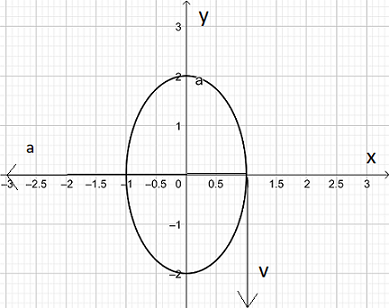# The position vector of a particle moving in the xy-plane is given by vector r of t equals sin of...

## Question:

The position vector of a particle moving in the {eq}xy {/eq}-plane is given by {eq}\vec{r}(t)=\langle \sin(2t), 2 \cos(2t) \rangle, \space 0 \le t \le \pi. {/eq}

i) Sketch this plane curve.

ii) Find the velocity and acceleration vectors and sketch them for {eq}t=\pi/4. {/eq}

iii) Is the particle's acceleration vector orthogonal to its velocity vector at the point where {eq}t=pi/8. {/eq} Show your work.

## Graph of a Parametric Curve:

A parametric curve within the x-y plane is described by the couple of equations

{eq}x=x(t) \\ y=y(t) {/eq}

with t being the parameter.

The graph of the curve is found by plotting the point (x,y) when the parameter t varies.

We are given the parametric curve described by the position vector

{eq}\vec{r}(t)=\langle \sin(2t), 2 \cos(2t) \rangle, \space 0 \le t \le \pi. {/eq}

i) A sketch the curve is obtained plotting points (x,y) as t varies in the given interval and is reported in the figure below.

ii) The velocity and acceleration vectors at {eq}t=\pi/4. {/eq} are calculated as follows

{eq}\vec{v}(t) =\vec{r'}(t)=\langle 2\cos(2t), -4 \sin(2t) \rangle \\ \vec{a}(t) =\vec{v'}(t)=\langle -4\sin(2t), -8 \cos(2t) \rangle \\ \Rightarrow \vec{v}(\pi/4) = \langle 0, -4 \rangle \\ \Rightarrow \vec{a}(\pi/4) = \langle -4, 0 \rangle. {/eq}

The vector are superimposed to the curve in the figure below.

iii) The particle's acceleration and velocity at {eq}t=\pi/8 {/eq} are equal to

{eq}\vec{v}(t) =\vec{r'}(t)=\langle 2\cos(2t), -4 \sin(2t) \rangle \\ \vec{a}(t) =\vec{v'}(t)=\langle -4\sin(2t), -8 \cos(2t) \rangle \\ \Rightarrow \vec{v}(\pi/8) = \langle \sqrt{2}, -2\sqrt{2} \rangle \\ \Rightarrow \vec{a}(\pi/8) = \langle -2\sqrt{2} , -4\sqrt{2} \rangle {/eq}

Since the scalar product between the last two vectors is different from zero,

the vectors are not orthogonal.